﻿ None Invasive Intraocular Pressure Control Simulation: PF-04475270 Case StudyAmerican Journal of Biomedical Engineering

p-ISSN: 2163-1050    e-ISSN: 2163-1077

2018;  8(1): 12-19

doi:10.5923/j.ajbe.20180801.02### None Invasive Intraocular Pressure Control Simulation: PF-04475270 Case Study

Omer Hamid1, 2

1Medical Equipment Tecnology Department, Sattam Bin Abdalaziz University, AlKharj, Kindom of Saudi Arabia

2Faculty of Engineering, Gezira University, Sudan

Correspondence to: Omer Hamid, Medical Equipment Tecnology Department, Sattam Bin Abdalaziz University, AlKharj, Kindom of Saudi Arabia.
 Email: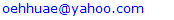Abstract

PF-04475270 is a hypotensive intraocular pressure (IOP) agent; its linearized 6th and reduced 3rd order Pharmacokinetics/Pharmacodynamics (PKPD) state space models were utilized in the design of model predictive control (MPC) systems. The PF-04475270 PKPD model is taken from the literature. In frequency domain the drug sixth order model is acting as a low pass filter with a cutoff frequency of 0.515 µHz. Kalman filter is used to obtain observer gain of the MPC and also to estimate the simulated noisy IOP. Assumption is made about the availability of: Stimulus responsive drug loaded hydrogel, contact lens IOP sensor and an embedded MPC system. One intentional model parameter doubling showed unbounded intraocular pressure open loop response but behaved well under the proposed linearized MPC; a preliminary test of sensitivity. Four eye drops kept IOP at a desired level for 48 hours. Continuous controlled drug delivery system designed and simulated based on PF-04475270 model has lowered IOP from 100% to 80% of its initial value despite of the added noise to the input and the output.

Keywords: Glaucoma, Model Predictive Control, Iris-Ciliary Body, Pharmacokinetics, Pharmacodynamics, Time-Frequency Drug Response

Cite this paper: Omer Hamid, None Invasive Intraocular Pressure Control Simulation: PF-04475270 Case Study, American Journal of Biomedical Engineering, Vol. 8 No. 1, 2018, pp. 12-19. doi: 10.5923/j.ajbe.20180801.02.

### 1. Introduction

Glaucoma is a group of eye ailments that leads to the malfunctioning of axons of ganglion cells and the ultimate loss of vision, if left untreated. Glaucoma is the main cause of permanent loss of sight . It is projected that the number of people contracting glaucoma worldwide will be 111.8 million in 2040, many of whom will be in Asia and Africa . The aqueous humor build up in the anterior chamber of the eye, causes high intraocular pressure. The aqueous humor, produced by the ciliary body, leaves the eye by passive flow via the trabecular meshwork into the episcleral veins or through the uveoscleral outflow pathway into the suprachoroidal space . Increased intraocular pressure is the only modifiable risk factor of glaucoma. The eye cornea is highly enervated region, eye drops initiate reflex and lacrimations that wash away most of the drug, only 5% or less bioavailability is usually guaranteed, indicating small residence time . The cornea presents stiff barrier to drugs penetration. Drugs must be hydrophobic to permeate the corneal epithelium and endothelium, and hydrophilic to permeate the corneal stroma [4, 5]. When the drug enters the anterior chamber it is carried by the aqueous flow away from the cite of intention, mostly the iris-ciliary body (icb). Patient adherence and compliance is a major problem in drug delivery especially for old people. The conventional ophthalmic drug dosing can be regarded as open loop control system vulnerable to disturbances. Off center drop dosing could introduce order of magnitude error in cornea concentration in the first early hours Maurice . IOP variation is a matter of concern following cataract surgery, as the intraocular pressure of a recovering patient can spike, leading to vision loss. Uneven drug staining leads to uneven drug diffusion and uneven drugs therapeutic effects. Eye drops is the first line of glaucoma treatment. Drugs, in form of eye drops, laser treatment or surgery are typical intervention methods widely available for controlling IOP. Commonly, single drug or combination of drugs is used . In case of patient incompliance or tolerance to drugs; laser or incision surgery is suggested. Drug delivery systems (as ocular inserts) capable of increasing the drug bioavailability and resident times were reviewed in . Molteno  in 1969, invented a glaucoma drainage device (GDD) made from a tube inserted into eye anterior chamber and draining into sub-conjunctival plate in response to an increase in IOP. The Ahmed Glaucoma Valve (AGV), an improved GDD , was available in 1996. Mechanical glaucoma drainage devices can be considered as closed loop IOP control systems. GDDs are the last options for refractory patients, although many patients benefited from them, they are invasive and have their own problems like endophthalmitisas as discussed in [11, 12]. In order to increase drug resident time and bioavailability, hydrogel contact lenses loaded with ophthalmic drugs were implicated in many trials for glaucoma treatment. With contact lenses the bioavailability has increased to 50% and the therapeutic effect has extended to days [13-16]. Although the advantage of using contact lenses controlled release is huge, the system is an open loop control system. Hydrogels can release drugs upon stimulation [17-20], a step forward in controlled drug release on demand. Brimonidine-loaded microspheres injected into the supraciliary space using a microneedle are able to reduce IOP for one month as an alternative to daily eye drops . Implanted drug device, controlled IOP for several weeks in rabbits [22, 23], but they are invasive and still open loop systems. Implanted IOP sensors for microsystems were suggested earlier . In order to control IOP using a non-invasive negative feedback closed loop control system, an IOP sensor must be available [25-29]. UNIST of Korea, 2018 , claimed making a LED-embedded contact Lens that can monitor glucose levels in tears and also suggested their invention may be used to monitor drugs in tears. If the LED is coupled with a photodetector, ocular fluorometry principles could be used to measure the concentration of drugs in the tears, cornea and the aqueous humour [31, 32]. This will provide more real signals in the negative feedback control system. One example of the early use of feedback technology to control a physiological parameter was seen in the case blood pressure control. Slate et al  in 1980 formulated a model to control blood pressure by infusing sodium nitroprusside. In this research an ophthalmic drug PF-04475270 (Pfizer) pharmacokinetic-pharmacodynamic (PKPD) equations in the time domain  were the basis equations for a model predictive control system as in Figure 1. The closed loop control system can include: A contact lens IOP sensor, an electric/photo responsive drug loaded hydrogel (reservoir), and an embedded microcontroller that receives the sensor output, stimulates the reservoir to release the drug on demand according to model predictive protocol. All these components can be mounted on a disposable contact lens. PF-04475270 causes IOP reduction via the activation of EP4 receptors [34, 35]. PF-04475270 is converted to CP-734432, shortly after dosing . CP- 734432 lowers IOP by increasing the conventional outflow via the trabecular meshwork. Invasive closed loop control system using a fluid pump was patented in . Up-to-date, there is no system that can control IOP automatically and noninvasively. The current work deals with the performance of the linearized PF-04475270 PKPD state space model in time and frequency domain. Also deals with the design and simulation of IOP model predictive control ophthalmic drug delivery system .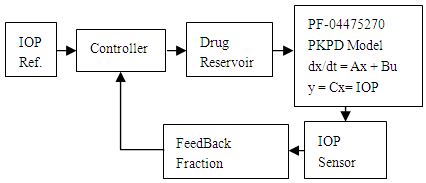Figure 1. A block diagram of IOP control system

### 2. Method

In this article, the model equations given in  are used to build a state space model. The equations' parameters are mainly transfer coefficients and elimination rates of the different model compartments representing different eye tissues. The set of equations describing the Pharmacokinetic-Pharmacodynamics model of the ophthalmic drug PF-04475270 , were linearized by Taylor's series ignoring the higher order terms (see Appendix). The linearized perturbation equations of the drug amount in different eye tissues and IOP response were formulated as state space model: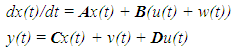(1)
where x represents a vector of state, perturbations , A is the dynamic matrix, B is input matrix, C the output matrix, and D is the feed-through matrix, y is the state open loop output and in this case it represents the IOP response to the drug dosing u(t) input, w(t), v(t) represent input and output random noise correspondingly. The A, B, C and D matrices are given in the Appendix, where the model rates are given /hr.
The state space model is an open loop control system, digitized at rate of one sample per hour. The model is simulated in Matlab environment (version 2015) in open and closed loop situations. MPC augmented PF-04475270 system is proposed for multiple dosing and an observer MPC based system is simulated for continuous dosing. The general structure of the proposed closed loop MPC system looks the same as given in [37, 39]; with random noise w(t) added to the input and v(t) to the output. The input noise w(t) could represent precorneal drug concentration variation due to reflex tearing and any possible disturbances of drug release or delivery mechanism. The output noise v(t) simulates the IOP sensor noise, and the endogenous biological noise due to neuron auto-regulation.

### 3. Results

The model is tested with one pulse (one eye drop) and the result is shown in Figure 2.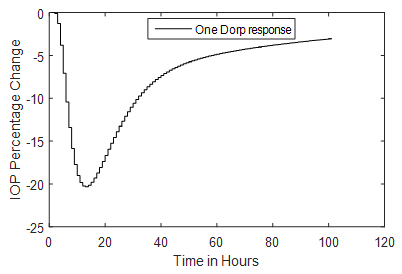Figure 2. A simulated one drop and IOP response of the linearized model
In frequency domain the model has six negative real poles with the most dominant pole (1/τ) at -0.010373 rad/hr. The model has one zero at-0.029. The model is controllable and observable as confirmed by Matlab instructions. The model has low pass frequency response shown in Figure 3, it has gain of 17.6 dB at 0.010 µHz and –3dB gain at 0.515 µHz.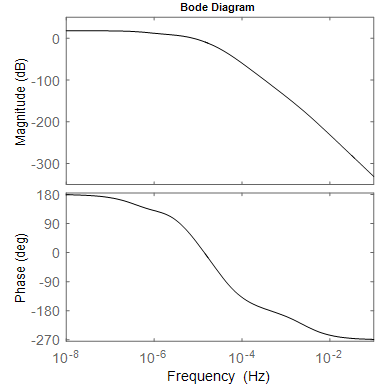Figure 3. Frequency characteristics of the model: magnitude and phase response, with gain of 17.6 dB at 0.010 µHz and –3dB point at 0.515 µHz
The model response under parameter variations is investigated by doubling kin, (Figure 4) which is the zero order rate constant for the buildup of IOP response , the response is unbounded. Using the three most dominant poles, the linearized sixth order PF-04475270 model is reduced to a third order model.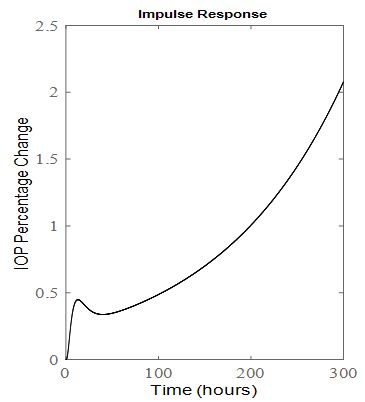Figure 4. Impulse response of the six order system when kin is doubled
The impulse(A,B,C,D) Matlab instruction calculates the unit response of PKPD PF-04475270 state space model as described by A, B, C and D matrices. The sixth and the third order models responses are shown in Figure 5. The third order system has -3dB point at 1880µHz, much faster than the sixth order model.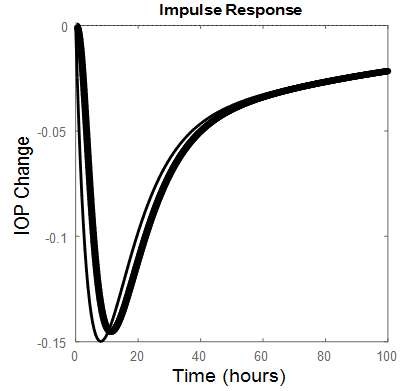Figure 5. Third order (thin line), Sixth order (thick line) impulse responses

#### 3.1. Multiple Dosing

A model predictive control (MPC) system was designed ; to adjust the future IOP responses. An augmented model was constructed. A prediction horizon of 48 (2 days), a control horizon of 4 (4-drops/4-hours) and a set point of -20% were used. The results are shown in Figure 6a. for six order MPC system. When kin is doubled, the result is presented in Figure 6b.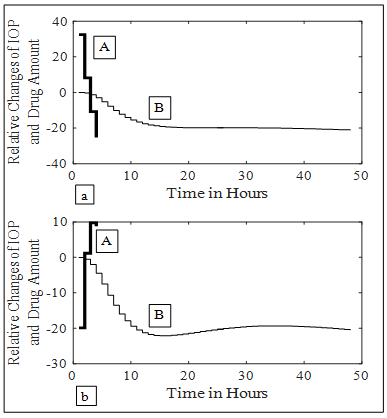Figure 6. MPC IOP control, A represents relative drug changes produced by the controller, B represents coressponding IOP four drops responses. (a) kin = 24.023. (b) kin= 48.046

#### 3.2. Continuous Dosing

A receding horizon and an observer for state estimation were employed to control the IOP at a set point of -20% for continuous controlled drug release. The gain of the observer was obtained by using Kalman filter. Also Kalman filter is used to estimate the noisy IOP as shown for the sixth order system in Figure 7. A noise of covariance Q =0.1, is added to the input of the system as process noise and a noise of covariance R = 10, is added to the IOP sensor output. Continuous drug dosing hour by hour; simulates drug release from a zero order contact lens hydrogel reservoir. Figure 7a shows the IOP control when the sixth order open loop system is stable i.e. kin=24.03. Figure 7b is the corresponding Kalman filer output. Figures 7c and 7d show IOP results at kin = 48.06. Appropriate selection of prediction horizon 12, just before it becomes unbounded, (Figure 4), has resulted in stable MPC response in Figure 7c. Similar results were obtained for the third order system (Figure 8).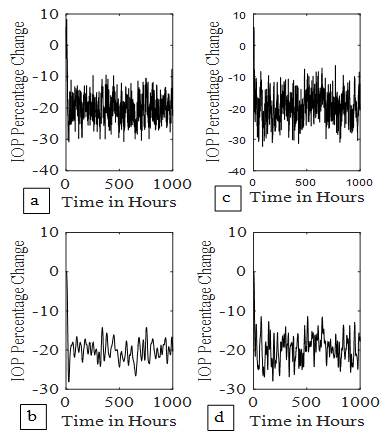Figure 7. IOP control by continuous dosing. (7a) 6th order system control at kin = 24.023, (7b) its Kalman filter output. (7c) 6th order system control at kin = 48.046, (7d) its Kalman filter output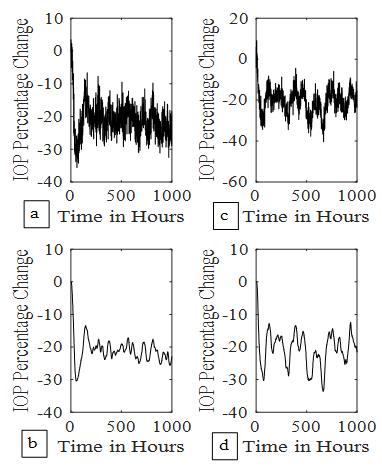Figure 8. IOP control by continuous dosing. (8a) 3rd order system control at kin = 24.023, (8b) its Kalman filter output. (8c) 3rd order system control at kin = 48.046, (8d) its Kalman filter output
The standard deviation (std) of noisy IOP percentage change (Kalman filter output) after some settling time is presented in Table 1, for two values of kin.
 Table 1. Effect of kin on IOP control. The standard deviation (std) of the Kalman estimate of IOP percentage change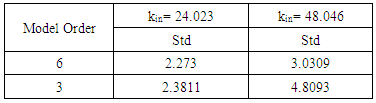### 4. Discussion

PF-04475270 PKPD formulated state space model IOP pulse response, reaches a desired level in 11.5 hours (Figure 2) resulting in frequency response passband of 0.515 µHz (Figure 3). If PF-04475270 is prescribed in the evening [34, 35], its maximum effect will appear in the morning where the IOP circadian is maximum. The circadian fundamental frequency is about 11.6 µHz, beyond the drug passband frequency, and can easily be filtered out by taking this drug which is behaving as a low pass filter having cutoff frequency = 0.515 µHz. The doubling of kin, has pushed the most dominant pole to the right hand side of the s-plane, an indication of instability as shown by the one drop response in Figure 4. for a six order model. Unknown diurnal, nocturnal IOP variability, episcleral venous pressure elevation, angle closure, and after surgery IOP fluctuation can be related to kin variations. The rigid eye globe, pain sensation and medication, put a limit to unbounded IOP in Figure 4; but before reaching that limit the ganglion cells will be damaged irreversibly.
A reduced third order model of the drug responds faster than the six order (Figure 5), a benefit in term of how quickly the IOP is eased, but the model become noisy with wider bandwidth (1880 µHz) compared to 0.515 µHz for the sixth order model.
Conventional one eye drop per day reduces IOP temporarily even in closed loop MPC system and IOP wonders again back towards its initial state [37, 39]. The minimum number of drops that can keep the IOP at its set level for long 48 hours (prediction horizon) was found to be 4 drops given in four consecutive hours (control horizon) as shown in Figure 6a. The augmented MPC system works , even under large single parameter variation (doubling of kin as shown in Figure 6a. The response of the closed loop is well behaved which indicates the eigen values of the closed loop system are within the unit circle, although the open loop system is not stable for large kin values. IOP fluctuation, transient and surge can be predicted and brought under control, because the model response is slow and works in micro-Hertz region.
The hour-by-hour dosing (continuous) system designed and simulated based on PF-04475270 model has lowered IOP from 100% to 80% of its initial value despite of the added noise to the input and the output (Figure 7-8). The system was found to be sensitive to input noise w(t), this is why the noise covariance made low (Q =0.1). The errors discussed by Maurice  in ocular fluorometry claimed variation in sodium fluorescein in eye tissue; by order of magnitude and can be lumped here as a single input source of noise. The system is to some extend immune to output noise v(t) (Q(t)=10). Both six (Figure 7) and third (Figure 8) order systems were stable, even when kin is doubled. The six order system noise performance (Table 1) is better than the third order system due to the bandwidth difference. The standard deviation (Table 1) of IOP change, after it settled to around -20%, is doubled when kin is doubled. The third order system implementation is cost effective.

### 5. Conclusions

An ophthalmic IOP reduction drug (PF-04475270) model is investigated in open loop and in closed loop MPC system for non-invasive regulation of IOP. Assuming one genuine parameter variation; the MPC is able to keep the IOP under control and reduces the future risk of high transient IOP and the consequent retinal injury.
Future work:
- do sensitivity study for all parameters and perhaps using Monte-Carlo method.
- find how eye pathology modify model parameters.
- deal with stability issues of the model predictive control system in which PKPD model acting as a plant.

### Appendix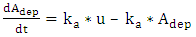(A.1)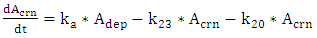(A.2)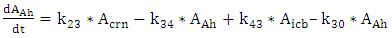(A.3)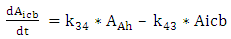(A.4)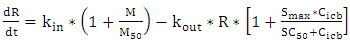(A.5)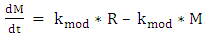(A.6)
As quoted from .
Where:
Adep= deposited amount of drug on the eye
u = drug input, usually as eye drops (not in the original model (see )
Acrn = amount of drug in the cornea
Aah = amount of drug in aqueous humor
Aicb = amount of drug in the iris ciliary body (icb) and its concentration Cicb
R = drug response taken as intraocular pressure (IOP)
M = model feedback modulator
At t = 0:
Cicb = 0; dR/dt = 0; M = M0, R = R0 and
kin = R0*kout/[1 + M0/M50] from Ref. 
R0 = M0 = 100%.
The rest of the symbols are model rates and coefficients given below (see Ref.  for more details):
ka = 0.188; k20= 0.834; k23 = 0.004; k30 = 0.171; k34 = 21.7; k43 = 34.8; kout = 0.653; Smax = 0.287; SC50 = 0.674; kmod = 0.029; M50 = 58.2; kin = 24.023; V= 0.05 (icb distribution volume).
State assignments:
x1 =Adep, x2 =Acrn, x3 =Aah, x4 =Aicb= Cicb*V, x5 = R =IOP, and x6 =M.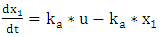(A.1.1)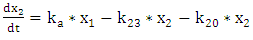(A.2.2)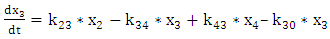(A.3.3)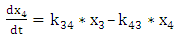(A.4.4)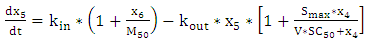(A.5.5)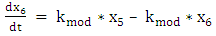(A.6.6)
x = [x1 x2 x3 x4 x5 x6]T, Transpose
In the steady state (ss): x = [0 0 0 0 100 100]T and the states time derivatives = 0. From (A1.1)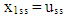(A.7)
From (A.2.2), it is possible to show, after some algebraic manipulations: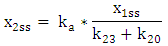(A.8)
From (A.3.3), it is possible to show, after some algebraic manipulations: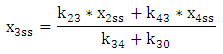(A.9)
From (A.4.4)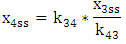(A.10)
From (A.5.5), it is possible to show, after some algebraic manipulations: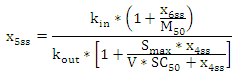(A.11)
From (A.6.6)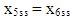(A.12)
It would be better to express all the steady states in terms of x1ss or uss. Using equations (A.8), (A.9) and (A.10), then: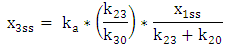(A.13)
Replace x3ss in (A.10)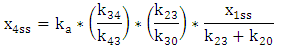(A.14)
Using equations (A.11) and (A.12) and after some manipulations, it is possible to show that x6ss=x5ss: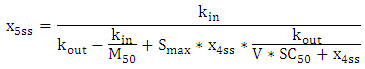(A.15)
If the drug is applied as a drop its concentration in the steady state or at infinite time becomes zero. And from the above equations x1ss = x2ss = x3ss= x4ss = 0.

### Linearization

Assuming Taylor's approximation: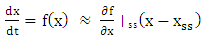(A.16)
The partial derivatives are evaluated at the steady state and the system is expressed in terms of small perturbation:
x = (x – xss) and u = (u - uss).
Taking the partial derivatives of the RHS of equations (A.1.1) to (A.6.6) w.r.t. x1…..x6 results in a system matrix A with elements ranging from a11 to a66: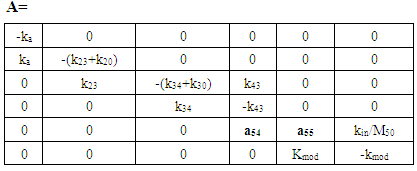(A.17)
The elements: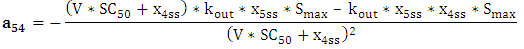(A.18)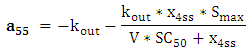(A.19)
The matrix B represents the single input (drug dosing), and the matrix C represents the single measurable output (IOP):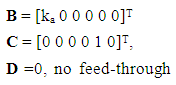(A.20)
And the state space equations given by: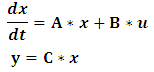(A.21)
In the steady state uss = 0 = x1ss; i.e. the eye drop as a pulse dies out after a long time.
From equation (A.14):
x4ss = 0;
Equation (A.15) becomes: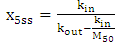(A.22)
The element a54 in equation (A.18) after putting the exact values of the various parameters and making x4ss = 0, becomes in term of kin: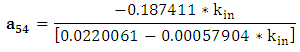(A.23)
This will allow the study of the PKPD model under one parameter variations, a56 also depends on kin.
Element a55 in equation (A.19) becomes:
a55 = -kout, when x4ss = 0.
At the given model parameters  the system dynamic matrix becomes: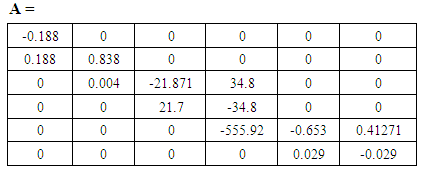The transfer function (which is the gain relationship in the frequency domain between the output IOP and the input u ) of the six order system is given by: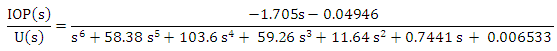(A.24)
With a zero at -0.029 and six poles at -0.010373, -0.67163, -0.1052, -56.566, -0.838, -0.188, and a gain = -1.7061. The poles are in rad/hr. The most dominant pole = -0.010373 has shifted to 0.0072571 in the right hand side of the s-domain, when kin is doubled (instability).
Using the most three dominant poles-0.010373, -0.1052, and -0.188. The PKPD system is reduced to a third order system with TF: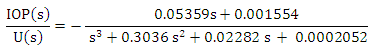(A.25)
- Author Statement Research funding: Author states no funding involved.
- Conflict of interest: Author states no conflict of interest.